Symmetry Worksheets

Symmetry worksheets consist of a variety of skills to understand the lines of symmetry in different shapes. Exercises to identify and draw the lines of symmetry, complete the shapes, count the lines of symmetry in each shape, to identify symmetrical or asymmetrical shapes and to determine the perimeter of shapes are given here for practice.

Recognizing lines of symmetry

Each worksheet shows eight different shapes through which a dotted line is drawn. Identify if the dotted line is a line of symmetry.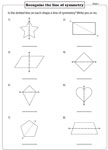Drawing a line of symmetry: Shapes

Three exclusive worksheets to identify and draw a line of symmetry on each shape are given here.Drawing a line of symmetry: Real-life objects

Draw a line of symmetry for each real-life objects. Some objects may have more than one line of symmetry.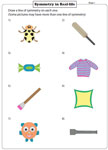Lines of symmetry: More than one line

Shapes with more than one line of symmetry are given. Determine and draw lines of symmetry on each shape and write down the number of lines of symmetry seen.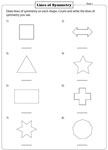Complete the mirror image: Standard

One-half of symmetrical shapes are shown. Draw the other half of the mirror image.Complete the mirror image: Grid

One-half of real-life objects are drawn in the grids. Complete the second half of each symmetrical shape.Cut-paste activity: Symmetric / Asymmetric

Cut out each shape from the bottom and paste them in the appropriate category (symmetric or asymmetric shapes).Cut-paste activity: Complete the shape

A symmetrical half of the shapes are shown. The other half of each shape is given below. Cut out the other half and paste them to complete the shape.Symmetry on English letters

Identify if the English letters shown are cut at the line of symmetry in part 1. Draw lines of symmetry on the given English letters in part 2.Unfold the mystery

Each folded paper has a cutout region. Choose the exact shape obtained at the center when the paper is unfolded.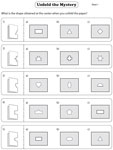Perimeter of symmetrical shapes

Each worksheet shows eight symmetrical shapes with all the possible lines of symmetry. Find the perimeter of each symmetrical shape using the information given.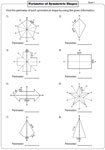U.S. Customary Units:

Metric Units:

Obtain the entire collection of worksheets on symmetry in a jiffy!

Related Worksheets